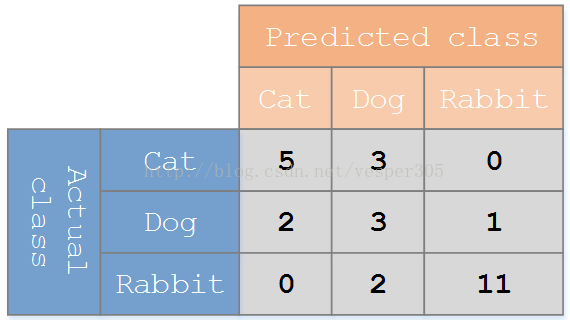# sklearn.metrics中的评估方法介绍（accuracy_score, recall_score, roc_curve, roc_auc_score, confusion_matrix）

1. accuracy_score

• 形式

sklearn.metrics.accuracy_score(y_true, y_pred, normalize=True, sample_weight=None)

normalize：默认值为True，返回正确分类的比例；如果为False，返回正确分类的样本数

• 示例

>>>import numpy as np
>>>from sklearn.metrics import accuracy_score
>>>y_pred = [0, 2, 1, 3]
>>>y_true = [0, 1, 2, 3]
>>>accuracy_score(y_true, y_pred)
0.5
>>>accuracy_score(y_true, y_pred, normalize=False)
2

1. recall_score

• 形式

klearn.metrics.recall_score(y_true, y_pred, labels=None, pos_label=1,average='binary', sample_weight=None)

macro：计算二分类metrics的均值，为每个类给出相同权重的分值。当小类很重要时会出问题，因为该macro-averging方法是对性能的平均。另一方面，该方法假设所有分类都是一样重要的，因此macro-averaging方法会对小类的性能影响很大。

weighted:对于不均衡数量的类来说，计算二分类metrics的平均，通过在每个类的score上进行加权实现。

micro：给出了每个样本类以及它对整个metrics的贡献的pair（sample-weight），而非对整个类的metrics求和，它会每个类的metrics上的权重及因子进行求和，来计算整个份额。Micro-averaging方法在多标签（multilabel）问题中设置，包含多分类，此时，大类将被忽略。

samples：应用在multilabel问题上。它不会计算每个类，相反，它会在评估数据中，通过计算真实类和预测类的差异的metrics，来求平均（sample_weight-weighted）

averageaverage=None将返回一个数组，它包含了每个类的得分.

• 示例：

>>>from sklearn.metrics import recall_score
>>>y_true = [0, 1, 2, 0, 1, 2]
>>>y_pred = [0, 2, 1, 0, 0, 1]
>>>recall_score(y_true, y_pred, average='macro')
0.33...
>>>recall_score(y_true, y_pred, average='micro')
0.33...
>>>recall_score(y_true, y_pred, average='weighted')
0.33...
>>>recall_score(y_true, y_pred, average=None)
array([1.,  0., 0.])

1. roc_curve

ROC曲线指受试者工作特征曲线/接收器操作特性(receiver operating characteristicROC)曲线,是反映灵敏性和特效性连续变量的综合指标,是用构图法揭示敏感性和特异性的相互关系，它通过将连续变量设定出多个不同的临界值，从而计算出一系列敏感性和特异性。ROC曲线是根据一系列不同的二分类方式（分界值或决定阈），以真正例率（也就是灵敏度）（True Positive Rate,TPR）为纵坐标，假正例率（1-特效性）（False Positive Rate,FPR）为横坐标绘制的曲线。

ROC观察模型正确地识别正例的比例与模型错误地把负例数据识别成正例的比例之间的权衡。TPR的增加以FPR的增加为代价。ROC曲线下的面积是模型准确率的度量AUCArea under roccurve）。

TPR = TP /（TP + FN）  （正样本预测结果数 / 正样本实际数）

FPR = FP /（FP + TN） （被预测为正的负样本结果数 /负样本实际数）

• 形式：

sklearn.metrics.roc_curve(y_true,y_score, pos_label=None, sample_weight=None, drop_intermediate=True)

“Score”表示每个测试样本属于正样本的概率。

• 示例

>>>import numpy as np
>>>from sklearn import metrics
>>>y = np.array([1, 1, 2, 2])
>>>scores = np.array([0.1, 0.4, 0.35, 0.8])
>>>fpr, tpr, thresholds = metrics.roc_curve(y, scores, pos_label=2)
>>>fpr
array([0. ,  0.5,  0.5, 1. ])
>>>tpr
array([0.5,  0.5,  1. , 1. ])
>>>thresholds
array([0.8 ,  0.4 ,  0.35, 0.1 ])
>>>from sklearn.metrics import auc
>>>metrics.auc(fpr, tpr)
0.75 

1. Auc

• 形式：

sklearn.metrics.auc(x, y, reorder=False)

1. roc_auc_score

• 形式：

sklearn.metrics.roc_auc_score(y_true, y_score, average='macro', sample_weight=None)

average : string, [None, ‘micro’, ‘macro’(default), ‘samples’, ‘weighted’]

• 示例：

>>>import numpy as np
>>>from sklearn.metrics import roc_auc_score
>>>y_true = np.array([0, 0, 1, 1])
>>>y_scores = np.array([0.1, 0.4, 0.35, 0.8])
>>>roc_auc_score(y_true, y_scores)
0.75

1. confusion_matrix
• 用一个例子来理解混淆矩阵：• 形式：

sklearn.metrics.confusion_matrix(y_true, y_pred, labels=None, sample_weight=None)

labels：混淆矩阵的索引（如上面猫狗兔的示例），如果没有赋值，则按照y_true, y_pred中出现过的值排序

• 示例

>>>from sklearn.metrics import confusion_matrix
>>>y_true = [2, 0, 2, 2, 0, 1]
>>>y_pred = [0, 0, 2, 2, 0, 2]
>>>confusion_matrix(y_true, y_pred)
array([[2,0, 0],
[0, 0, 1],
[1, 0, 2]])

>>>y_true = ["cat", "ant", "cat", "cat","ant", "bird"]
>>>y_pred = ["ant", "ant", "cat", "cat","ant", "cat"]
>>>confusion_matrix(y_true, y_pred, labels=["ant", "bird","cat"])
array([[2,0, 0],
[0, 0, 1],
[1, 0, 2]])

04-204838

#### sklearn.metrics.precision_recall_curve & sklearn.metrics.average_precision_score计算方法05-318540

#### 使用sklearn accuracy_score,f1_score,roc_auc_score,recall_score,precision_score对模型进行评估

03-211万+

#### sklearn.metrics中的评估方法介绍(accuracy_score, recall_score, roc_curve, roc_auc_score, confusion_matrix)

09-082万+

#### sklearn计算准确率和召回率----accuracy_score、metrics.precision_score、metrics.recall_score

06-062万+

#### sklearn学习：roc_auc曲线和 metrics.roc_auc_score

08-281万+

#### sklearn.metrics.roc_curve用法

12-038633

#### Python3写精确率(precision)、召回率(recall)以及F1分数(F1_Score)

04-17422

#### sklearn.metrics中的评价方法 precision_score,recall_score,F分数（f1_score，f_beta）和accuracy_score©️2020 CSDN 皮肤主题: 终极编程指南 设计师: CSDN官方博客点击重新获取扫码支付1.余额是钱包充值的虚拟货币，按照1:1的比例进行支付金额的抵扣。
2.余额无法直接购买下载，可以购买VIP、C币套餐、付费专栏及课程。余额充值